Limits calculation formulas

The limit of the constant equals to this constant: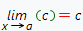(c - constant)

The limit of the sum equals to the sum of the limits: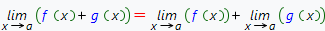The limit of the difference equals to the difference of the limits: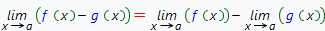The limit of the multiplication equals to the multiplication of the limits: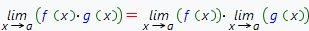The limit of the division equals to the division of the limits: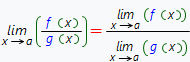if the condition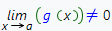is hold

The power function limit: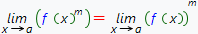(m - natural number)

The root function limit: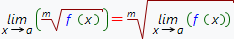(m - natural number)

The main limits:

The first wonderful limit: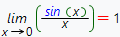(x - angle in radians)

The second wonderful limit: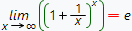Other useful limits formulas: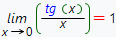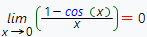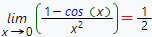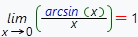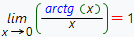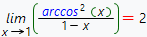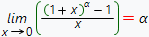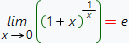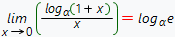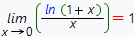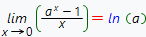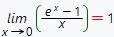infinitesimal

Infinitesimal equivalence:

As x → 0 , the following functions are equivalent:

x ~ sin(x) ~ arcsin(x) ~ tg(x) ~ arctg(x) ~ ln(1+x)

To find the limit of the division of two infinitesimal functions, they can be substituted by their equivalents: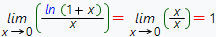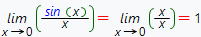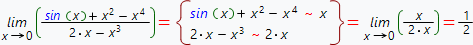To calculate the limits you can use our online calculator.